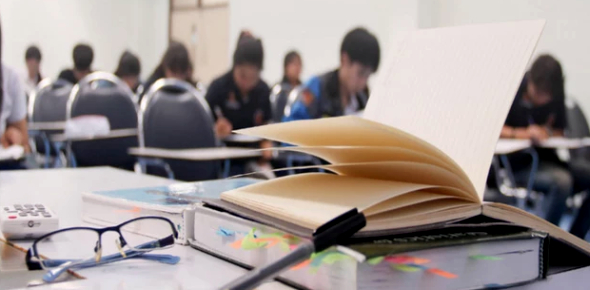Science Online Exam: MCQ Quiz!

45 Questions | Total Attempts: 114Settings.

• 1.
A steel bar of 5 mm is heated from 15c to 40c and it is free to expand. The bar will induce
• A.

No stress

• B.

Shear stress

• C.

Tensile stress

• D.

Compressive stress

• 2.
The stress-induced in a body, when suddenly loaded, is __________ the stress-induced when the same load is applied gradually.
• A.

Equal to

• B.

One-half

• C.

Twice

• D.

Four times

• 3.
The deformation per unit length is called:
• A.

Tensile stress

• B.

Compressive stress

• C.

Shear stress

• D.

Strain

• 4.
Two closely coiled helical springs 'A' and 'B' are equal in all respects but the number of turns of spring 'A' is half that of spring 'B' The ratio of deflections in spring 'A' to spring 'B' is
• A.

1/8

• B.

1/4

• C.

1/2

• D.

2

• 5.
When a rectangular beam is loaded transversely, the maximum compressive stress is developed on the:
• A.

Top layer

• B.

Bottom layer

• C.

Neutral axis

• D.

Every cross-section

• 6.
The friction experienced by a body, when in motion, is known as:
• A.

Rolling friction

• B.

Dynamic friction

• C.

Limiting friction

• D.

Static friction

• 7.
If the resultant of two equal forces have the same magnitude as either of the forces, then the angle between the two forces is:
• A.

60°

• B.

90°

• C.

120°

• D.

Either A or C

• 8.
The unit of angular acceleration is:
• A.

N-m

• B.

M/s

• C.

M/s2

• D.

• 9.
Work done is said to be zero, when
• A.

Some force acts on a body, but displacement is zero

• B.

No force acts on a body but some displacement takes place

• C.

Both (A) & (B)

• D.

None of the above

• 10.
If the masses of both the bodies, as shown in the below figure, are reduced to 50 percent, then tension in the string will be:
• A.

Same

• B.

Half

• C.

Double

• D.

None of the above

• 11.
Geometric primitives include shapes such as ________.
• A.

Boxes

• B.

Cylinders

• C.

Wedges

• D.

All of the above

• 12.
This is the term for the range of tightness or looseness resulting from the allowances and tolerances in mating parts:
• A.

Limits

• B.

Fit

• C.

Specifications

• D.

Allowance

• 13.
This means that a feature of a finished product contains the maximum amount of material permitted by the toleranced dimensions for that feature:
• A.

Maximum material condition

• B.

Machined material condition

• C.

Maximum machined indication

• D.

Machine mark indication

• 14.
A cylinder can be created by drawing a rectangular shape than the ________ tool.
• A.

Revolve

• B.

Sweep

• C.

Extrude

• D.

None of the above

• 15.
CAD programs that incorporate parametric modeling utilize a system in which the dimensions control the ________.
• A.

Size and shape of the model features

• B.

Perspective of the model

• C.

Shading used to render the model

• D.

All of the above

• 16.
In one dimensional flow, the flow
• A.

• B.

Takes place in straight line

• C.

Takes place in curve

• D.

Takes place in one direction

• 17.
Euler's equation in the differential form for the motion of liquids is given by
• A.

Dp/p + g.dz + v.dv = 0

• B.

Dp/p - g.dz + v.dv = 0

• C.

ρ.dp + g.dz + v.dv = 0

• D.

ρ.dp - g.dz + v.dv = 0

• 18.
According to equation of continuity,
• A.

W1a1 = w2a2

• B.

W1v1 = w2v2

• C.

A1v1 = a2v2

• D.

A1/v1 = a2/v2

• 19.
The loss of head due to friction in a pipe of uniform diameter in which a viscous flow is taking place, is (where RN = Reynold number)
• A.

1/RN

• B.

4/RN

• C.

16/RN

• D.

64/RN

• 20.
The pressure less than atmospheric pressure is known as
• A.

Suction pressure

• B.

Vacuum pressure

• C.

Negative gauge pressure

• D.

All of these

• 21.
Which of the following statement is wrong?
• A.

The heat transfer in liquid and gases takes place according to convection.

• B.

The amount of heat flow through a body is dependent upon the material of the body.

• C.

The thermal conductivity of solid metals increases with rise in temperature.

• D.

Logarithmic mean temperature difference is not equal to the arithmetic mean temperature difference.

• 22.
In a refrigerating machine, heat rejected is __________ heat absorbed.
• A.

Equal to

• B.

Less than

• C.

Greater than

• D.

Two times

• 23.
The amount of radiation mainly depends upon the
• A.

Nature of the body

• B.

Temperature of the body

• C.

Type of surface of the body

• D.

All of these

• 24.
The thickness of thermal and hydrodynamic boundary layer is equal if Prandtl number is
• A.

Equal to one

• B.

Greater than one

• C.

Less than one

• D.

Equal to Nusselt number

• 25.
The subcooling in a refrigeration cycle
• A.

Does not alter C.O.P.

• B.

Increases C.O.P.

• C.

Decreases C.O.P.

• D.

None of these

Related TopicsBack to top